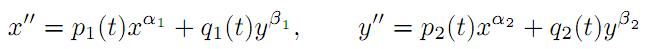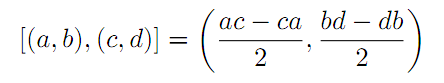The Australian Journal of Mathematical Analysis and Applications

 Home News Editors Volumes RGMIA Subscriptions Authors Contact

ISSN 1449-5910

Sorry death is imminent for file: ../public_html/searchroot/files/tex/v19n2/v19i2p4.tex
You searched for niu
Total of 43 results found in site

21: Paper Source PDF document

Paper's Title:

On Some Mapping Properties of Möbius Transformations

Author(s):

Nihal Yilmaz Özgür

Balkesir University, Faculty of Arts and Sciences, Department of Mathematics,
10145 Balkesir,
TURKEY
nihal@balikesir.edu.tr
URL
: http://w3.balikesir.edu.tr/~nihal/

Abstract:

We consider spheres corresponding to any norm function on the complex plane and their images under the Möbius transformations. We see that the sphere preserving property is not an invariant characteristic property of Möbius transformations except in the Euclidean case.

3: Paper Source PDF document

Paper's Title:

Hyperbolic Barycentric Coordinates

Author(s):

Abraham A. Ungar

Department of Mathematics, North Dakota State University,
Fargo, ND 58105,
USA
Abraham.Ungar@ndsu.edu
URL
: http://math.ndsu.nodak.edu/faculty/ungar/

Abstract:

A powerful and novel way to study Einstein's special theory of relativity and its underlying geometry, the hyperbolic geometry of Bolyai and Lobachevsky, by analogies with classical mechanics and its underlying Euclidean geometry is demonstrated. The demonstration sets the stage for the extension of the notion of barycentric coordinates in Euclidean geometry, first conceived by Möbius in 1827, into hyperbolic geometry. As an example for the application of hyperbolic barycentric coordinates, the hyperbolic midpoint of any hyperbolic segment, and the centroid and orthocenter of any hyperbolic triangle are determined.

3: Paper Source PDF document

Paper's Title:

Construction of a Frame Multiresolution Analysis on Locally Compact Abelian Groups

Author(s):

R. Kumar and Satyapriya

Department of Mathematics,
Kirori Mal College,
University of Delhi,
Delhi,
India.
E-mail: rajkmc@gmail.com

Department of Mathematics,
University of Delhi,
Delhi,
India.
E-mail: kmc.satyapriya@gmail.com

Abstract:

The frame multiresolution analysis (FMRA) on locally compact Abelian groups has been studied and the results concerning classical MRA have been worked upon to obtain new results. All the necessary conditions, which need to be imposed on the scaling function φ to construct a wavelet frame via FMRA, have been summed up. This process of construction of FMRA has aptly been illustrated by sufficient examples.

2: Paper Source PDF document

Paper's Title:

A Wallis Type Inequality and a Double Inequality for Probability Integral

Author(s):

Jian Cao, Da-Wei Niu and Feng Qi

School of Mathematics and Informatics,
Henan Polytechnic University, Jiaozuo City,
Henan Province, 454010,
China
21caojian@163.com

School of Mathematics and Informatics,
Henan Polytechnic University, Jiaozuo City,
Henan Province, 454010,
China
nnddww@tom.com

Research Institute of Mathematical Inequality Theory,
Henan Polytechnic University, Jiaozuo City,
Henan Province, 454010,
China
qifeng@hpu.edu.cn
fengqi618@member.ams.org
qifeng618@hotmail.com
qifeng618@msn.com
qifeng618@qq.com
URL: http://rgmia.vu.edu.au/qi.html

Abstract:

In this short note, a Wallis type inequality with the best upper and lower bounds is established. As an application, a double inequality for the probability integral is found.

2: Paper Source PDF document

Paper's Title:

A Study of the Effect of Density Dependence in a Matrix Population Model

Author(s):

N. Carter and M. Predescu

Department of Mathematical Sciences,
Bentley University,
Waltham, MA 02452,
U.S.A.
ncarter@bentley.edu
mpredescu@bentley.edu

Abstract:

We study the behavior of solutions of a three dimensional discrete time nonlinear matrix population model. We prove results concerning the existence of equilibrium points, boundedness, permanence of solutions, and global stability in special cases of interest. Moreover, numerical simulations are used to compare the dynamics of two main forms of the density dependence function (rational and exponential).

2: Paper Source PDF document

Paper's Title:

Preserver of Local Spectrum of Skew-product Operators

Author(s):

1Department of Mathematics,
College of Sciences,
University of Yasouj,
Yasouj, 75918-74934,
Iran.
E-mail: r.parvinian@yu.ac.ir

2Department of Mathematics,
College of Sciences,
University of Yasouj,
Yasouj, 75918-74934,
Iran.

3Department of Mathematics,
Ghor Institute of higher education,
Afghanistan.
E-mail: jumapazhman@gmail.com

Abstract:

Let H and K be infinite-dimensional complex Hilbert spaces, and B(H) (resp. B(K)) be the algebra of all bounded linear operators on H (resp. on K). For an operator T B(H) and a vector h H, let σT(h) denote the local spectrum of T at h. For two nonzero vectors h0 H and k0 K, we show that if two maps φ1 and φ2 from B(H) into B(K) satisfy

σφ1(T)φ2(S)*(k0)= σTS*(h0})

for all T, S B(H), and their range containing all operators of rank at most two, then there exist bijective linear maps P : H K and Q : K H such that φ1(T) = PTQ and φ2(T)* =Q-1T*P-1 for all T B(H). Also, we obtain some interesting results in this direction.

1: Paper Source PDF document

Paper's Title:

The successive approximations method and error estimation in terms of at most the first derivative for delay ordinary differential equations

Author(s):

Alexandru Mihai Bica

Department of Mathematics,
Str. Armatei Romane no.5,
Romania
smbica@yahoo.com

Abstract:

We present here a numerical method for first order delay ordinary differential equations, which use the Banach's fixed point theorem, the sequence of successive approximations and the trapezoidal quadrature rule. The error estimation of the method uses a recent result of P. Cerone and S.S. Dragomir about the remainder of the trapezoidal quadrature rule for Lipchitzian functions and for functions with continuous first derivative.

1: Paper Source PDF document

Paper's Title:

On the Generalized Stability and Asymptotic Behavior of Quadratic Mappings

Author(s):

Hark-Mahn Kim, Sang-Baek Lee and Eunyoung Son

Department of Mathematics
Chungnam National University
Daejeon, 305-764,
Republic of Korea

hmkim@cnu.ac.kr

Abstract:

We extend the stability of quadratic mappings to the stability of general quadratic mappings with several variables, and then obtain an improved asymptotic property of quadratic mappings on restricted domains.

1: Paper Source PDF document

Paper's Title:

Fixed Points and Stability of the Cauchy Functional Equation

Author(s):

Choonkil Park and Themistocles M. Rassias

Department of Mathematics, Hanyang University,
Seoul 133-791,
Republic of Korea

Department of Mathematics, National Technical University of Athens,
Zografou Campus, 15780 Athens,
Greece

baak@hanyang.ac.kr
trassias@math.ntua.gr

Abstract:

Using fixed point methods, we prove the generalized Hyers-Ulam stability of homomorphisms in Banach algebras and of derivations on Banach algebras for the Cauchy functional equation.

1: Paper Source PDF document

Paper's Title:

A Note On The Global Behavior Of A Nonlinear System of Difference Equations

Author(s):

Norman H. Josephy, Mihaela Predescu and Samuel W. Woolford

Department of Mathematical Sciences,
Bentley University,
Waltham, MA 02452,
U.S.A.

mpredescu@bentley.edu
njosephy@bentley.edu
swoolford@bentley.edu

Abstract:

This paper deals with the global asymptotic stability character of solutions of a discrete time deterministic model proposed by Wikan and Eide in Bulletin of Mathematical Biology, 66, 2004, 1685-1704. A stochastic extension of this model is proposed and discussed. Computer simulations suggest that the dynamics of the stochastic model includes a mixture of the dynamics observed in the deterministic model.

1: Paper Source PDF document

Paper's Title:

A Differential Sandwich Theorem for Analytic Functions Defined by the Generalized Sălăgean Operator

Author(s):

D. Răducanu and V. O. Nechita

Faculty of Mathematics and Computer Science,
"Transilvania" University Braşov
Str. Iuliu Maniu 50, 500091 Braşov,
Romania

Faculty of Mathematics and Computer Science,
"Babeş-Bolyai" University Cluj-Napoca,
Str. M. Kogalniceanu 1, 400084 Cluj-Napoca,
Romania
URL: http://math.ubbcluj.ro/~vnechita/
vnechita@math.ubbcluj.ro

Abstract:

We obtain some subordination and superordination results involving the generalized Sălăgean differential operator for certain normalized analytic functions in the open unit disk. Our results extend corresponding previously known results.

1: Paper Source PDF document

Paper's Title:

Asymptotic Analysis of Positive Decreasing Solutions of a Class of Systems of Second Order Nonlinear Differential Equations in the Framework of Regular Variation

Author(s):

Jaroslav Jaroš, Kusano Takaŝi, Tomoyuki Tanigawa

Department of Mathematical Analysis and Numerical Mathematics,
Faculty of Mathematics, Physics and Informatics,
Comenius Universiy, 842 48 Bratislava,
Slovakia.

E-mail: ksntksjm4@gmail.com

Professor Emeritus at: Hiroshima University,
Department of Mathematics, Faculty of Science,
Higashi-Hiroshima 739-8526,
Japan.

E-mail: jaros@fmph.uniba.sk

Department of Mathematics, Faculty of Education,
Kumamoto University, Kumamoto 860-8555,
Japan.

E-mail: tanigawa@educ.kumamoto-u.ac.jp

Abstract:

The system of nonlinear differential equationsis under consideration, where αi and βi are positive constants and pi(t) and qi(t) are continuous regularly varying functions on [a,). Two kinds of criteria are established for the existence of strongly decreasing regularly varying solutions with negative indices of (A) with precise asymptotic behavior at infinity. Fixed point techniques and basic theory of regular variation are utilized for this purpose.

1: Paper Source PDF document

Paper's Title:

On the Hyers-Ulam Stability of Homomorphisms and Lie Derivations

Author(s):

Department of Mathematics, Payame Noor University,
P.O. Box: 19395-3697, Tehran,
Iran.

Abstract:

Let A be a Lie Banach*-algebra. For each elements (a, b) and (c, d) in A2:= A * A, by definitions

(a, b) (c, d)= (ac, bd),
|(a, b)|= |a|+ |b|,
(a, b)*= (a*, b*),

A2 can be considered as a Banach*-algebra. This Banach*-algebra is called a Lie Banach*-algebra whenever it is equipped with the following definitions of Lie product:for all a, b, c, d in A. Also, if A is a Lie Banach*-algebra, then D: A2→A2 satisfying

D ([ (a, b), (c, d)])= [ D (a, b), (c, d)]+ [(a, b), D (c, d)]

for all \$a, b, c, d∈A, is a Lie derivation on A2. Furthermore, if A is a Lie Banach*-algebra, then D is called a Lie* derivation on A2 whenever D is a Lie derivation with D (a, b)*= D (a*, b*) for all a, b∈A. In this paper, we investigate the Hyers-Ulam stability of Lie Banach*-algebra homomorphisms and Lie* derivations on the Banach*-algebra A2.

1: Paper Source PDF document

Paper's Title:

Introducing the Dorfmanian: A Powerful Tool for the Calculus Of Variations

Author(s):

Olivier de La Grandville

Department of Management Science and Engineering,
Stanford University,
475 Via Ortega, Stanford, CA 94305,
U. S. A.

E-mail: odelagrandville@gmail.com

Abstract:

We show how a modified Hamiltonian proposed by Robert Dorfman  to give intuitive sense to the Pontryagin maximum principle can be extended to easily obtain all high-order equations of the calculus of variations. This new concept is particularly efficient to determine the differential equations leading to the extremals of functionals defined by n-uple integrals, while a traditional approach would require -- in some cases repeatedly -- an extension of Green's theorem to n-space.
Our paper is dedicated to the memory of Robert Dorfman (1916 - 2002).

1: Paper Source PDF document

Paper's Title:

The Jacobson Density Theorem for Non-Commutative Ordered Banach Algebras

Author(s):

Kelvin Muzundu

University of Zambia,
Deparment of Mathematics and Statistics,
P.O. Box 32379, Lusaka,
Zambia.
E-mail: kmzundu@gmail.com

Abstract:

The Jacobson density theorem for general non-commutative Banach algebras states as follows: Let π be a continuous, irreducible representation of a non-commutative Banach algebra A on a Banach space X. If x1,x2,...,xn are linearly independent in X and if y1,y2,...,yn are in X, then there exists an a A such that π(a)xi=yi for i=1,2,...,n. By considering ordered Banach algebras A and ordered Banach spaces X, we shall establish an order-theoretic version of the Jacobson density theorem.

1: Paper Source PDF document

Paper's Title:

On the Oldest Problem in the Calculus of Variations: A New Message from Queen Dido

Author(s):

Olivier de La Grandville

Faculty of Economics,
Goethe University Frankfurt,
Theodore Adorno Platz 4, 60323 Frankfurt,
Germany.
E-mail: odelagrandville@gmail.com

Abstract:

We consider the problem of finding the optimal curve of given length linking two points in a plane such as it encloses a maximal area. We show that if the curve is not described by a single-valued function, its determination does not necessarily imply to work with a parametric representation of the curve. We show that a simpler approach is at hand -- and, who knows? -- this might well be the method Queen Dido used.

Search and serve lasted 1 second(s).